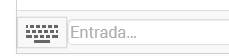# Triangles- Classification

Topic:
Triangles

Can you build an equilateral triangle?

Try typing in the input: D=(0,0) (aperte ENTER) E=(2,0) (aperte ENTER) F=(1,sqrt(3)) (press ENTER)* What can you see? * SQRT is the square root